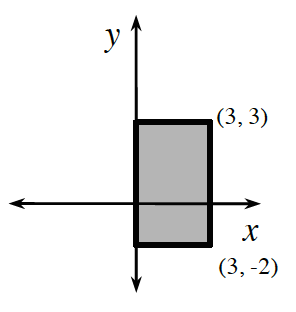### Home > CCA2 > Chapter 2 > Lesson 2.1.4 > Problem2-58

2-58.

Examine the diagram at right. Imagine spinning the rectangle around the $y$-axis. Think of a rectangular flap attached to the $y$-axis so that the rectangle will revolve around the $y$-axis.

1. Draw the resulting shape.

After the rotation, the resulting shape is a cylinder.

2. Find the volume of this shape.

Use the formula for volume of a cylinder, $V=\pi r^{2}h.$